Standard

# Functions

## A Function is Special

A function is a type of equation.

A function has special rules it must follow:

In a function there can ONLY be ONE output(y) for every input (x).

Just like I stated before, a Function is Special! 🙂

• It must work for every possible input value
• And it has only one relationship for each input value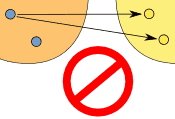THIS IS A FUNCTION!                  THIS IS NOT A FUNCTION!

### Example: y = x²

FUNCTIONCould also be written as a table:

X: x Y: x2
3 9
1 1
0 0
4 16
-4 16

It is a function, because:

• Every element in X is related to Y
• No element in X has two or more relationships
• The X values DO NOT repeat

So it follows the rules.

(Notice how both 4 and -4 relate to 16, which is allowed since 4 and -4 are two different numbers.)

### Example: This relationship is not a function:

NOT A FUNCTIONThis is not a function, for these reasons:

• Value “3” in X has no Y
• Value “4” in X has no Y
• Value “5” is related to more than one value in Y
• REMEMBER THE X VALUE CANNOT REPEAT

# Unit Rate

Unit rates are about the amount for 1 unit.

We use Ratio Tables to help solve for rates.
Our Ratio table looks like a tic-tac-toe table.We MUST label our table to know where to put our information

Ex. Bob drives 100 miles in 5 hours. How many miles does he travel every hour?Now we fill in the information that is given.

Bob drives 100 miles in 5 hours. How many miles does he travel every hour?Once we have entered what was given we need to see what the question is asking us.

Ex. Bob drives 100 miles in 5 hours. How many miles does he travel every hour?

Ask yourself, is the problem asking how many hours it takes for 1 mile or how many miles for 1 hour???????

If you said how many miles for 1 hour, you are correct!

So now we enter that into our Ratio Table.The x is the unknown. This is what we are solving for.To solve for x, we multiply 100 and 1. Then divide by the number left over which is 5.

We end up with x = 20.

So Bob drives 20 miles per hour.

Click here to watch a video with a different way to find unit rate.

Test yourself on unit rates here!

# Unit 4: Slope and Y-Interecept

Standard

### Slope Intercept Form:      y = mx + b

The variable m is the slope, it explains the steepness of a line of how slanted the line is.

The variable b is the y-intercept, this is where the line crosses or intersects the y-axis.

https://www.desmos.com/calculator

http://www.shmoop.com/video/slope-intercept-form/

# Slope: m

Slope is how steep a straight line is.
When finding the slope from a line on a graph we use the method of rise over run .
• Rise is how far up
• Run is how far alongYou can also think of it as the change in y over the change in x.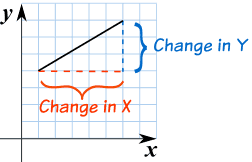EXAMPLE #1:The slope here is 4/6 which can simplify to 2/3.
EXAMPLE #2:In this example the slope is 3/5.

# Practice Problems

TEST YOUR SELF ON LINES HERE!!!!

https://my.hrw.com/wwtb/api/viewer.pl

# Y Intercept: b

Y intercept is where a straight line crosses the Y axis of a graph.

### Example: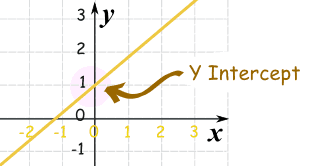In the above diagram the line crosses the Y axis at 1.

So the Y intercept is equal to 1.

# Slope Intercept Form

### y = mx + b

m = Slope (how steep the line is)

b = the Y Intercept (where the line crosses the Y axis)\### How do you find “m” and “b”?

• b is easy: just see where the line crosses the Y axis.
• m (the Slope) needs some calculation. Remember to write slope as a fraction.

## Example 1)The fastest and easiest thing to find first when looking at a graph is the y-intercept (b).

Here we see that the line crosses the y-axis at positive 1.

So, b = 1 .

Now to find the slope (m) we will use rise over run:

• Rise is how far up
• Run is how far along

In this example the rise is 2 and the run is 1.

So, m = 2/1 .

Now that I know m = 2/1 and b = 1 I can plug them into the equation for slope intercept form y = mx + b.

y = (2/1) x + 1

^^^^^ This is the equation of the line.

Standard

# Equations

What is an equation?

Examples: 4 + 3  = 7        or        3x + 5 = 10

An equation is a number sentence. We call it an equation because it has an equal sign.

## The 5 Steps to Writing an Equation or Inequality

#### Step 5.Write the equations

Don’t forget our cool ‘dance’ we did to remember this!

WRITING EQUATIONS PRACTICE PROBLEMS:

## With Equations, Inequalities and Expressions we always want to combine like terms 1st!

Here is an example on how to do that:Once all like terms have been combined then we can solve.

# Solving Equations with ModelsMODELING EQUATIONS PRACTICE PROBLEMS:

# Solving Equations Algebraically

### Solve √(x/2) = 3

 Start With √(x/2) = 3 Square both sides: x/2 = 32 32 = 9: x/2 = 9 Multiply both sides by 2: x = 18

And the more “tricks” and techniques you learn the better you will get.

Here is an example of how we solved equations in class:SOLVING EQUATIONS (with variables on both sides PRACTICE PROBLEMS:

Click here or here to practice Solving Equations online and get automatic feedback (it grades it)! 🙂

# Simple vs. Compound  Interest

### Introduction to interest:

http://www.mathsisfun.com/money/interest.html

### SIMPLE INTEREST

I = Prt

• I = interest owed  [\$] (this is ONLY the interest borrowed)
• P = amount borrowed (called “Principal”)  [\$]
• r = interest rate   [%] (you have to divide the percent by 100)  For information On Percents click here!
• t = time    [years]

Simple interest is money you can earn by investing some money (the principal). The interest (percent) is the rate that makes the money grow!

### COMPOUND INTEREST

A = P(1+r)^t

• A = All of it / Actual / total amount owed (this amount includes the interest and the principal)   [\$]
• P = amount borrowed (called “Principal”)    [\$]
• r = interest rate     [%]
• t = time    [years]

Compound interest is very similar to simple interest. The difference is that compound interest grows much faster! The reason it grows faster is because the interest (percent) has an exponent.

********** MAKE SURE TO READ THE QUESTION AND SEE EXACTLY WHAT IT IS ASKING DOES IT JUST WANT THE INTEREST OR THE TOTAL (All of it) ???????? *************************

For information on compound interest click here.

SIMPLE INTEREST PRACTICE PROBLEMS:

Click here or here to practice Simple Interest online and get automatic feedback (it grades it)! 🙂

COMPOUND INTEREST PRACTICE PROBLEMS:

Click here or here to practice Compound Interest online and get automatic feedback (it grades it)! 🙂

Standard

# Mean

The mean is the average of the numbers.

It is easy to calculate: add up all the numbers, then divide by how many numbers there are.

# Absolute Value

Absolute Value means …

… only how far a number is from zero:“6” is 6 away from zero, and “−6” is also 6 away from zero. So the absolute value of 6 is 6, and the absolute value of −6 is also 6

# Mean Absolute Deviation

Mean absolute deviation (MAD) is about calculating the average distance from the mean.

Click here to watch a video from our 8th Grade Math textbook that goes in to detail and explain how to calculate the MAD.

MEAN ABSOLUTE DEVIATION:

The average/mean distance from the average/mean is the MAD.

Yes, we use “mean” twice: Find the mean … use it to work out distances … then find the mean of those!

### Three steps:

• 1. Find the mean of all values
• 2. Find the distance of each value from that mean (subtract the mean from each value, ignore negative signs)
• 3. Then find the mean of those distances

Like this:

### 3, 6, 6, 7, 8, 11, 15, 16

Step 1: Find the mean:

 Mean = 3 + 6 + 6 + 7 + 8 + 11 + 15 + 16 = 72 = 9 8 8

Step 2: Find the distance of each value from that mean:

Value Distance from 9
3 6
6 3
6 3
7 2
8 1
11 2
15 6
16 7

Which looks like this:Step 3. Find the mean of those distances:

 Mean Deviation = 6 + 3 + 3 + 2 + 1 + 2 + 6 + 7 = 30 = 3.75 8 8

So, the mean = 9, and the mean deviation = 3.75

It tells us how far, on average, all values are from the middle.

In that example the values are, on average, 3.75 away from the middle.

### For deviation just think distance

MEAN ABSOLUTE DEVIATION Practice Problems:

## Random Sample

This is where everyone has an equal and fair chance of being selected.

Standard

# REAL NUMBERS

In this unit we went over Real Numbers and the classification system that is set up with Real Numbers. Here is the Rational Numbers Poster we created in classWe used Nesting boxes to demonstrate how the sub groups are inside each other.

 Description of Each Set of Numbers Natural The natural numbers (also known as counting numbers) are the numbers that we use to count. It starts with 1, followed by 2, then 3, and so on. 1, 2, 3, 4… Whole The whole numbers are a slight “upgrade” of the natural numbers because we simply add the number zero to the current set of natural numbers. Think of whole numbers as natural numbers and the number zero. 0, 1, 2, 3, 4… **Remember a hole in the ground is shaped like the number zero!** Integers The integers include all whole numbers together with the “opposites” of the natural numbers (their negatives). … -4, -3, -2, -1, 0, 1, 2, 3, 4 … Rational The rational numbers are numbers which can be expressed as ratio of integers. That means, if we can write a given number as a fraction where the numerator and denominator are both integers; then it is a rational number. Caution: The denominator cannot equal to zero. Rational numbers can also appear in decimal form. If the decimal number either terminates or repeats, then it is possible to write it as a fraction with an integer numerator and denominator. Thus, it is rational as well Irrational The irrational numbers are all numbers which when written in decimal form does not repeat and does not terminate. Real The real numbers includes both the rational and irrational numbers. Remember that under the rational number, we have the subcategories of integers, whole numbers and natural numbers.

# Ordering Numbers

Watch a video about ordering number here!

To order numbers there are a couple steps to follow.

1st: Convert all you numbers to decimals

2nd: Convert these to percents

3rd: Label a number line

4th: Put your numbers on the number line

## Ascending Order

To put numbers in order, place them from lowest (first) to highest (last).

This is called “Ascending Order”.

Example: Place 17, 5, 9 and 8 in ascending order.

• Answer: 5, 8, 9, 17

## Descending Order

Sometimes you want the numbers to go the other way, from highest down to lowest, this is called “Descending Order”.

Example: Place 17, 5, 9 and 8 in descending order.

• Answer: 17, 9, 8, 5

#### Click here  or here    or here   or here  or here to practice Ordering Numbers online and get automatic feedback (it grades it)! 🙂# Scientific Notation

Scientific notation is about writing really big and really small numbers in an equivalent form.

We normally write numbers in what we call STANDARD NOTATION, but we can also write this number in SCIENTIFIC NOTATION.

For example the number three hundred and twenty.

STANDARD:   320

SCIENTIFIC NOTATION: 3.2 × 102

## CONVERTING NUMBERS

When given a number in scientific notation you can easily convert the number to standard notation.

Example #1

Convert from Scientific Notation:  3.6 × 1012

to Standard Notation

Now, since the exponent on 10 is positive, I know they are looking for a LARGE number, so I’ll need to move the decimal point in the positive direction (to the right), in order to make the number LARGER. Since the exponent on 10 is “12“, I’ll need to move the decimal point twelve places over.

First, I’ll move the decimal point twelve places over. I make little loops when I count off the places, to keep track:Then I fill in the loops with zeroes: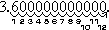In other words, the number is 3,600,000,000,000, or 3.6 trillion

Example #2

Convert 4.2 × 10–7 to standard notation.

Since the exponent on 10 is negative, I am looking for a small number. Since the exponent is a seven, I will be moving the decimal point seven places. Since I need to move the point to get a small number, I’ll be moving it to the left. The answer is 0.00000042

#### Click here to do Scientific Notation practice online and get automatic feedback (it grades it)! 🙂# 2015-2016 School Year Advisory Acceleration Expectations

Standard

The video below outlines the expectations for 8th Grade Math Advisory Acceleration.

Standard

# COMPARING COSTS

## 2 year/60 credit hours comparison of in-district/in-state tuition costs 2014-2015

ACC’s low tuition gives you the opportunity to pursue a college degree without breaking the bank. We’re the most affordable choice for college in the area.In class we used the ACC website and Cappex to compare college prices for tuition.

Tuition is a sum of money charged for teaching or instruction by a school, college, or university.

We viewed the video below and explored different Financial Aid options.

# Right Triangles and Pythagorean Theorem

Standard

The Pythagorean Theorem states that, in a right triangle, the two smaller squares made from the triangles legs add up to equal the biggest square made from the hypotenuse.The lengths of the legs are commonly labeled as a and b and the hypotenuse length is labeled as c.REMEMBER:

HYPOTENUSE: c is the longest side of the triangle

LEGS: a and b are the other two sides## What is the Pythagorean Theorem?

In short the Pythagorean Theorem can be explain with the formula below.

## a2 + b2 = c2

AGAIN, THIS IS ONLY TRUE FOR RIGHT TRIANGLES!

Here are other examples: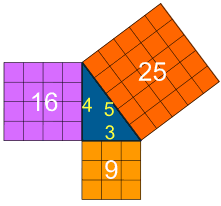When not knowing the Pythagorean Theorem can cost you \$15,000.

# Angle and Triangle Relationships

Standard

## Degrees

A degree is a unit of measurement used to measure angles.

### We can measure all Angles in Degrees.

There are 360 degrees in one Full Rotation (one
complete circle around

## The Degree Symbol: °

We use a little circle ° following the number to mean degrees.

For example 90° means 90 degrees

## Angles### In One Diagram

This diagram might make it easier to remember:

Also: Acute, Obtuse and Reflex are in alphabetical order.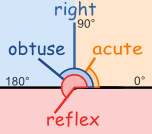# Supplementary Angles

Two Angles are Supplementary if they add up to 180 degrees.
 These two angles (140° and 40°) are Supplementary Angles, because they add up to 180°. Notice that together they make astraight angle.But the angles don’t have to be together. These two are supplementary because 60° + 120° = 180°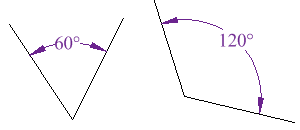# Complementary Angles

Two angles are Complementary when they
(a Right Angle).

 These two angles (40° and 50°) are Complementary Angles, because they add up to 90°.Notice that together they make a right angle.But the angles don’t have to be together. These two are complementary because 27° + 63° = 90°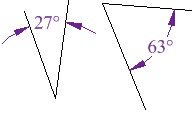# Interior Angle

### An Interior Angle is an angle inside a shape.# Exterior Angle

### The Exterior Angle is the angle between any side of a shape, and a line extended from the next side.# Angles On One Side of A Straight Line

### Angles on one side of a straight line will always add to 180 degrees.

If a line is split into 2 and you know one angle you can always find the other one.30° + 150° = 180°

# Interior Angles of Polygons

### An Interior Angle is an angle inside a shape.## Triangles

The Interior Angles of a Triangle add up to 180°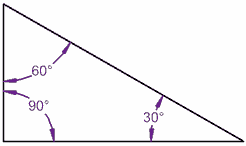### 80° + 70° + 30° = 180°

It works for this triangle!

Let’s tilt a line by 10° …

It still works, because one angle went up by 10°, but the other went down by 10°

(A Quadrilateral has 4 straight sides)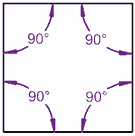### 80° + 100° + 90° + 90° = 360°

A Square adds up to 360°

Let’s tilt a line by 10° … still adds up to 360°!

 The interior angles in this triangle add up to 180° (90°+45°+45°=180°)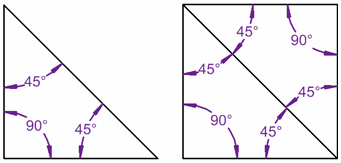… and for this square they add up to 360° … because the square can be made from two triangles!